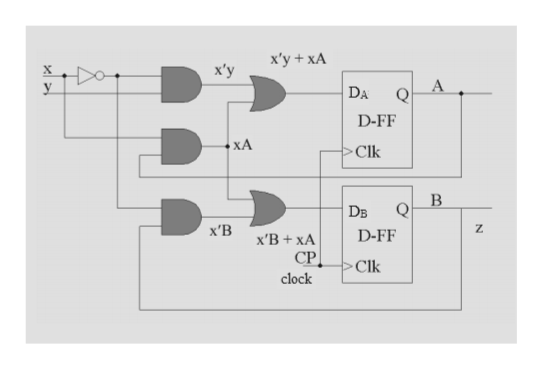# A sequential circuit with two D flip flops A and B, two inputs x and y and one output z is specified by the following next state and output equation:A(t+1) = x’y + xAB(t+1) = x’B + xAz = Ba) Draw the logic diagram of the circuitb) List the state table for the sequential circuitc) Draw the corresponding state diagram

Question
562 views

A sequential circuit with two D flip flops A and B, two inputs x and y and one output z is specified by the following next state and output equation:

A(t+1) = x’y + xA

B(t+1) = x’B + xA

z = B

a) Draw the logic diagram of the circuit

b) List the state table for the sequential circuit

c) Draw the corresponding state diagram

check_circle

Step 1

D flip flop means delay flip flop which provide delay for some time and give output same as input. Next state and input of flip flop are equal.

x and x are inputs and z is output.

DA= A* = x’y + xA

DB = B* =x’B + xA

z=A (output is independent of input)

A(t+1), B(t+1) are next states. In D flip flop to get the next states A(t+1), B(t+1) for A, B(present state), we have to give next state value to D of D flip flop. So sequential circuit will be:

Step 2

To get next state as A(t+1)= x’y + xA, B(t+1)= x’B + xA, we have to give them to DA, DB of D flip flop because for D flip flop whatever is given to DA, DB will appear at output A, B.help_outlineImage Transcriptioncloseх'у + хA х'у х у Q DA D-FF ХА Clk В Q DB Z х'В D-FF х'В + ХА СР. Clk clock fullscreen
Step 3

To get the state table first take present state from A B -> 00 to 11 and fill output z, A(t+...

### Want to see the full answer?

See Solution

#### Want to see this answer and more?

Solutions are written by subject experts who are available 24/7. Questions are typically answered within 1 hour.*

See Solution
*Response times may vary by subject and question.
Tagged in

### Computer Science